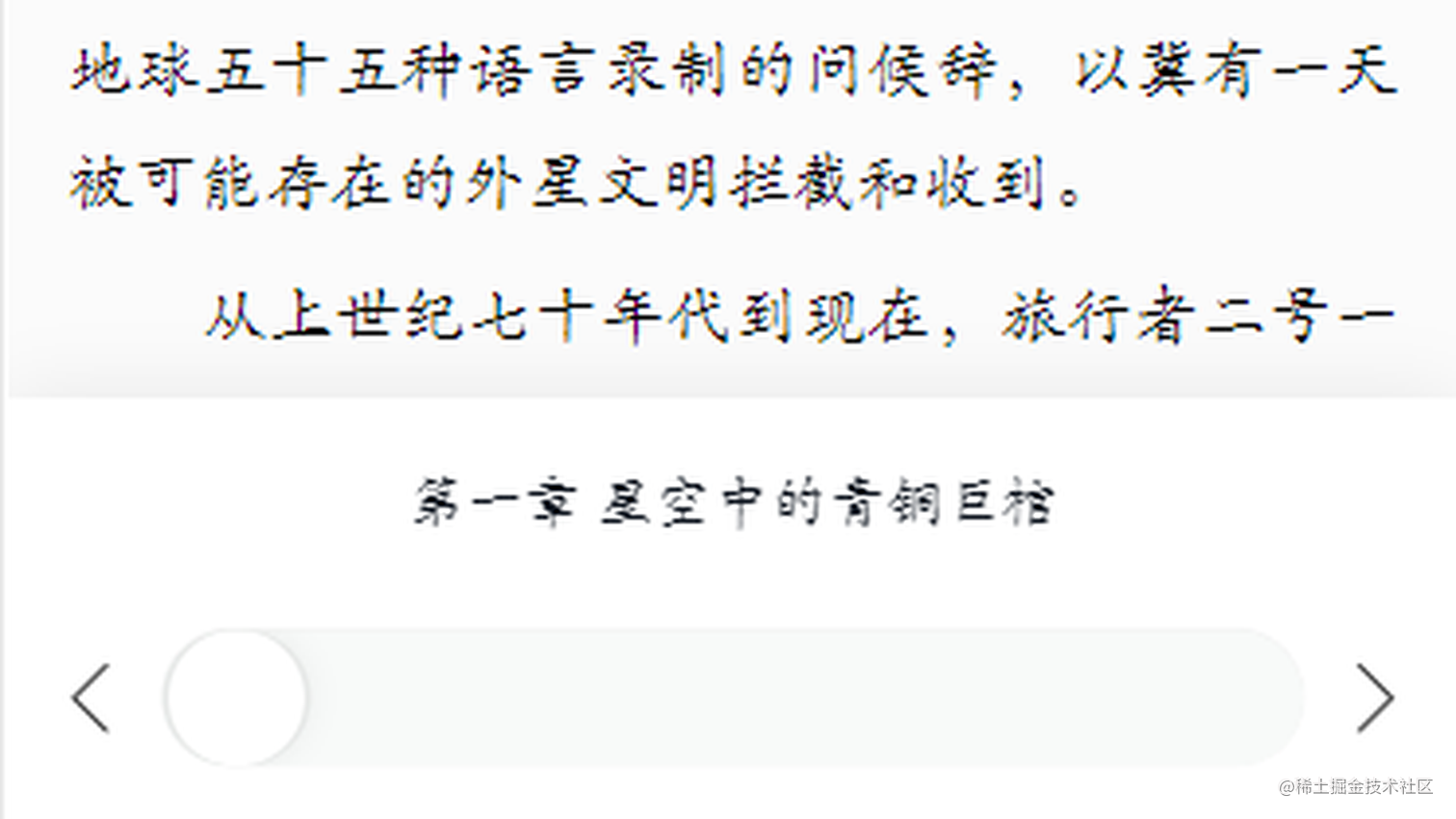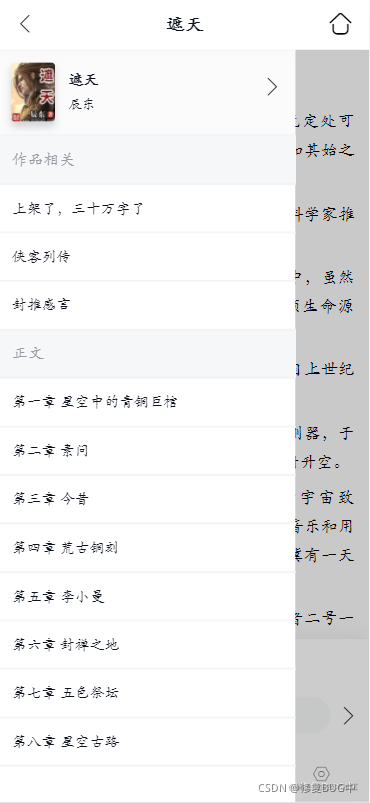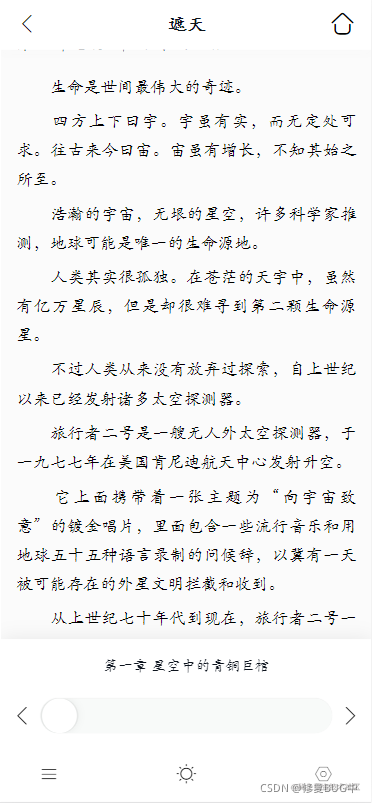# ❤️一文掌握HTML+CSS+JS开发小说阅读器❤️1. 阅读器功能划分及效果图
2. 拖动进度条切换章节
3. 上下滚动、左右滑动翻页
4. 其它模块`````` /**
* 拖动进度条
*/
let now = \$('#r-progress-now'); // 已拖动进度条
let bar = \$('#r-progress-bar'); // 背景进度条
\$('#r-progress').on('touchstart', function () { // 监听用户触发屏幕事件
let chapterId; // 记录章节ID
let _this 	   = this;
let width 	   = 60; // 进度条每次滑动值
let width_bar = bar.offsetWidth - this.offsetWidth - 3; // 背景进度条实际使用长度

_this.ontouchmove = function(ev) { // 监听圆点拖动事件
ev = ev || window.event;
let width_new = ev.changedTouches.clientX - width; // 圆点距离背景进度条左边距离值
if (width_new <= 0) { // 设置最小值
width_new = 0;
}

if (width_new >= width_bar) { // 设置最大值
width_new = width_bar;
}

let percent    = (width_new / width_bar).toFixed(2); // 计算当前拖动百分比
index          = parseInt(percent * menuLength); // 当前数组下标

// 更新章节ID、章节名及已拖动进度条的长度值

now.css('width', width_new + 'px');
};

_this.ontouchend = function() { // 监听用户离开屏幕事件

// 根据当前ID获取章节
changeChapter(chapterId);
}
});``````
/**
* 左右翻页
*/
let rContent = \$('#r-section'); // 阅读器盒子对象
let touch = {
startX: 0,
count: 0
};

/**
* 阅读器弹出操作栏或左右翻页效果
*/
rContent.on('touchstart', function(event){
// 当前点击距离屏幕的距离
touch.startX = event.targetTouches['pageX'];

if (touch.startX > contentWidth / 4 && touch.startX < (contentWidth * 3 / 4)) { // 弹出操作栏
\$('#r-footer').css('display', 'block');

// touch 和 click事件的相关冲突，有touch 就没有了，click功能，解决方法：去掉
event.preventDefault();
return false;
return;
}

if (changeKey === '1') { // 上下滚动
return;
}

if (touch.startX < contentWidth / 4 && touch.count <= 0) { // 当前为第一页
return;
}

if (touch.startX < contentWidth / 4 ) { // 向上翻页
touch.count --;
}

if (touch.startX > (contentWidth * 3 / 4)) { // 向下翻页
touch.count ++;
}

// 计算平移值，contentWidth 为屏幕的阅读器宽度，初始化时计算的
let translateWidth = -contentWidth * touch.count;
\$('#r-section').css('transform', 'translateX(' + translateWidth + 'px)');

});